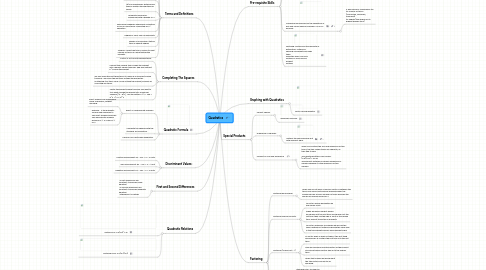または 登録 あなたのEメールアドレスで登録Quadratics## 2. Pre-requisite Skills

### 2.1. Multiply Polynomials

2.1.1. Consists of distributing two binomials into each other. As the first term in the bracket will multiply into the second bracket and so forth.

### 2.2. Distributing brackets and simplifying the expression

2.2.1. Distribution & Collecting Like Terms

2.2.1.1. Distributive property is used to expand algerbraic expressions

2.2.1.2. Once you have expanded you must simplify the expression by collecting like terms

2.2.1.3. If you are distributing with a non-constant term (i.e. A term with a variable), then the exponent laws apply

### 2.3. Classifying Polynomials by the separation of plus and minus signs for example: 2x+3 is a binomial.

2.3.1. A polynomial is classified by its:: Its number of terms (monomials, binomials, trinomials) Its degree (the degree of its highest degree term)

## 3. Special Products

### 3.1. Perfect Square:

3.1.1. squaring a binomial

### 3.2. Difference of Squares:

3.2.1. multiply the same binomial but with different signs

### 3.3. Product of Sum and Difference

3.3.1. When you multiply the sum and difference of two terms, the two middle terms are opposite, so they add to zero. The resulting pattern is as follows: (a-b)(a+b) = a2-b2 The product obtained is called a difference of squares because it is the difference of two squares.

## 8. Factoring

### 8.1. Factoring by grouping

8.1.1. When you do not have a common factor in between the terms you should factoring by grouping when the polynomial has an even number of terms because the groups are usually groups of 2.

### 8.2. Factoring Special Profucts

8.2.1. To factor special quadratics we use square roots.

8.2.2. When we have a perfect square we square root the first term and square root the last term then use the sign in front of the middle term and put those two in brackets.

8.2.3. To factor difference of squares we will use the same method but instead of having the same sign in the two brackets we will have different signs.

### 8.3. Factoring (a does not = 1)

8.3.1. To factor when A does not equal 1 the first thing we would do is multiply the first term into the last term.

8.3.2. Then we we would find the factors of the product and look at which factors add up to the middle term.

8.3.3. When that is done we would input the two factors and factor by grouping.

### 8.4. Factoring (a=1)

8.4.1. Standard form: a2+2ab+b2

8.4.2. Factored form: a(x-r)(x-s)

8.4.3. To factor when a=1 we usually take the factors of the the last term and see which two factors add up to the first term

### 8.5. Common Factors

8.5.1. Factor by grouping

8.5.2. Use Greatest Common Factor to simplify the polynomial